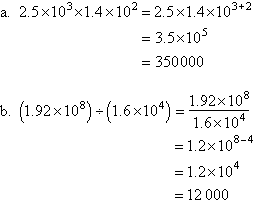Year 10 Interactive Maths - Second Edition

## Standard Form (Scientific Notation)

Astronomers, biologists, engineers, physicists and many others encounter quantities whose measures involve very small or very large numbers.  For example, the distance of the Earth from the Sun is approximately 144,000,000,000 metres and the distance that light will travel in 1 year is 5,870,000,000,000 metres.

It is sometimes tedious to write or work with such numbers.  This difficulty is overcome by writing such numbers in standard form.

E.g.  144,000,000,000 = 1.44 × 10¹¹
5,870,000,000,000 = 5.87 × 10¹²

If a quantity is written as the product of a power of 10 and a number that is greater than or equal to 1 and less than 10, then the quantity is said to be expressed in standard form (or scientific notation).

For example, 658 = 6.58 × 10²

###### Note:
• We have expressed 658 as a product of 6.58 and a power of 10.  Clearly, 6.58 is between 1 and 10.  So the standard form of 658 is 6.58 × 10².
• The decimal point is shifted to the left by 2 places, and 2 appears as the positive index in the power of 10.
###### In general:

In converting a number to standard form, if the decimal point is shifted to the left p places, then p appears as a positive index in the power of 10.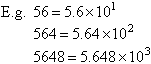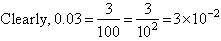###### Note:

The decimal point is shifted to the right by 2 places, and the negative of 2 appears as the index in the power of 10.

###### In general:

In converting a number to standard form, if the decimal point is shifted to the right p places, then the negative of p appears as a negative index in the power of 10.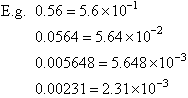#### Example 15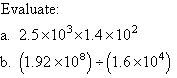##### Solution: